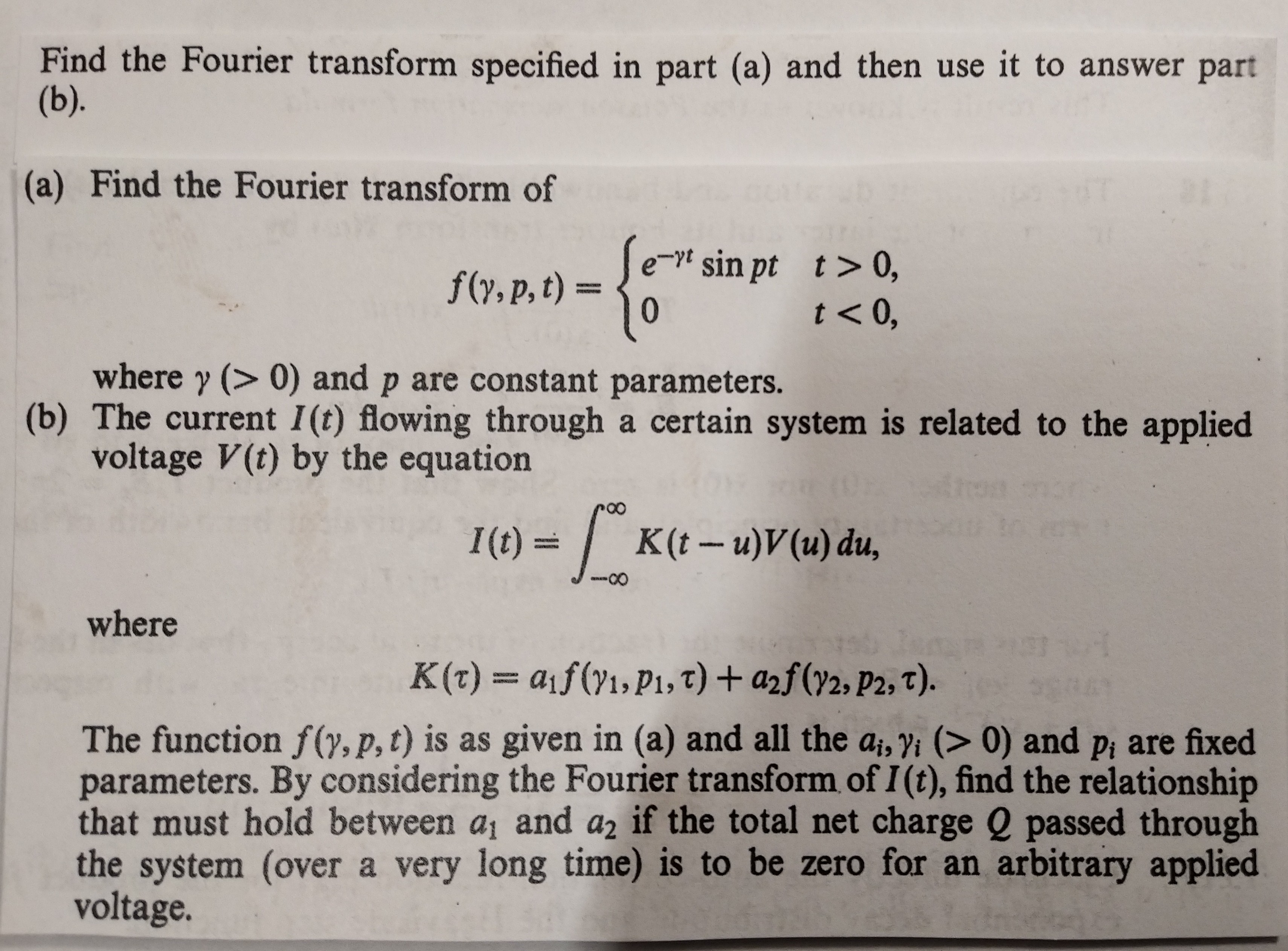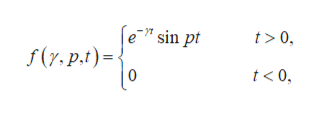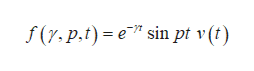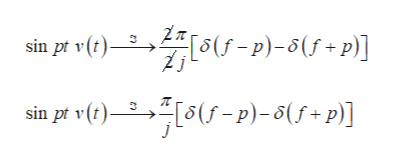Find the Fourier transform specified in part (a) and then use it to answer part(b).(a) Find the Fourier transform off(y, p, t)Jet sin pt t> 0,0'0>1where y (> 0) and p are constant parameters.(b) The current 1(t) flowing through a certain system is related to the appliedvoltage V(t) by the equationArosI(t)=K(t - u)V(u) du,wherenOrK(t) = aif (y1, Pi, T) + a2f(72, P2, T).The function f(y, p, t) is as given in (a) and all the ai, yi (> 0) and pi are fixedparameters. By considering the Fourier transform of I (t), find the relationshipthat must hold between ai and a2 if the total net charge Q passed throughthe system (over a very long time) is to be zero for an arbitrary appliedvoltage.

Questionhelp_outlineImage TranscriptioncloseFind the Fourier transform specified in part (a) and then use it to answer part (b). (a) Find the Fourier transform of f(y, p, t)Jet sin pt t> 0, 0 '0>1 where y (> 0) and p are constant parameters. (b) The current 1(t) flowing through a certain system is related to the applied voltage V(t) by the equation Aros I(t)= K(t - u)V(u) du, where nOr K(t) = aif (y1, Pi, T) + a2f(72, P2, T). The function f(y, p, t) is as given in (a) and all the ai, yi (> 0) and pi are fixed parameters. By considering the Fourier transform of I (t), find the relationship that must hold between ai and a2 if the total net charge Q passed through the system (over a very long time) is to be zero for an arbitrary applied voltage. fullscreen
Step 1

(a)

Consider the provided function.help_outlineImage Transcriptionclosee" sin pt t> 0 f(y,p.t) 0 t<0 fullscreen
Step 2

The provided function can be written as:help_outlineImage Transcriptionclosef (y.p.t) e sin pt v (t) fullscreen
Step 3

Now

...help_outlineImage TranscriptioncloseZ(p)-f+p)] sin pt v(t sin pt v(t) 5-p)-of+p)] fullscreen

Want to see the full answer?

See Solution

Want to see this answer and more?

Our solutions are written by experts, many with advanced degrees, and available 24/7

See Solution
Tagged in

Math